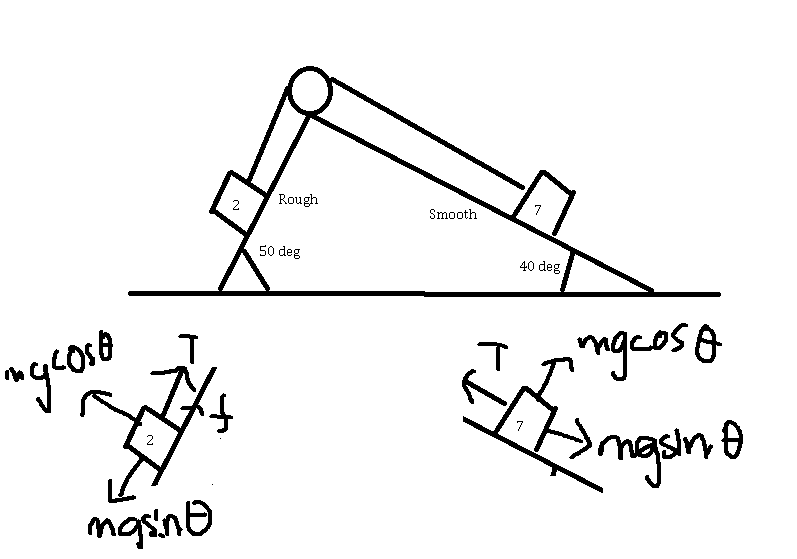# Acceleration of masses in a pulley system

• jisbon

#### jisbon

Homework Statement
2 masses on each side of the inclined plane. Coefficient of kinetic friction = 0.3, held by an inextensible string.
Masses of blocks as labelled on the diagram
Relevant Equations
-So I figured out the equation, but it is probably wrong because the answer doesn't tally.
Since the string is inextensible, I can assume that tension is the same for both sides, and acceleration for both masses is the same too So:
I can say that the acceleration of 2kg block =acceleration of 7kg block

$$\dfrac {7g\sin 40-T}{2+7}=\dfrac {T+\left( 0.3\right) \left( 2g\cos 50\right) -2g\sin 50}{7+2}$$

I got T = 29.36N, and used one of the equation to get my acceleration. However, it seems to be wrong :/

Is there something wrong with my equation? Thanks

•